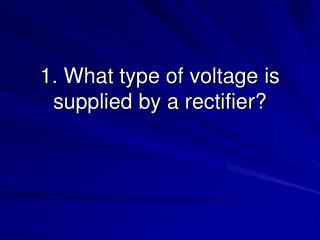# 1. What type of voltage is supplied by a rectifier? - PowerPoint PPT PresentationDownload Presentation1. What type of voltage is supplied by a rectifier?

1. What type of voltage is supplied by a rectifier?Download Presentation## 1. What type of voltage is supplied by a rectifier?

- - - - - - - - - - - - - - - - - - - - - - - - - - - E N D - - - - - - - - - - - - - - - - - - - - - - - - - - -
##### Presentation Transcript

1. 1. What type of voltage is supplied by a rectifier?

2. Pulsating DC voltage.

3. 2. What type of voltage is usually required by most electronic equipment?

4. Smooth DC voltage

5. To smooth out the pulsating DC voltage.

6. 4. What do you call the filter that uses a capacitor as the first component following the rectifier output?

7. Capacitor input filter.

8. 5. What does a capacitor oppose?

9. Change in voltage.

10. 6. What do you call the filter that uses a coil as the first component following the rectifier output?

11. Choke input filter.

12. 7. What does a choke filter oppose?

13. Change in current.

14. Low current applications.

15. 9. What is the symbol for pi?

16. 10. Which is the most common filter configuration found in power supplies?

17. A pi filter with a coil.

18. 11. What is a filter circuit?

19. It is a network of resistors and inductors or capacitors.

20. 12. What does “filter” mean in electronics?

21. To filter means to oppose or reject voltages and currents of certain frequencies while also offering little opposition to or passing of voltages and currents of other frequencies.

22. 13. What do filter circuits block?

23. Certain frequencies.

24. 14. What does an RL circuit contain?

25. Both resistance and inductance.

26. 15. What opposes current flow in an RL circuit?

27. Resistance and inductive reactance.

28. 16. What is impedance?

29. The total opposition to current flow in RL circuits.

30. 17. What do you call a filter circuit that contains resistance and capacitance?

31. An RC filter circuit.

32. 18. What is another term for “cut-off frequency”?

33. Half-power point.

34. 19. If input frequency decreases in an RL circuit, what happens to voltage across the resistor?

35. Voltage across the resistor increases.

36. 20. What is another name for a high-frequency discriminator?

37. A low-pass filter.

38. 21. What is a high-pass filter?

39. A filter that passes high frequencies and discriminates or blocks low frequencies.

40. 22. What is a low-pass filter?

41. A filter that passes low frequencies and discriminates or blocks the high frequencies.

42. 23. How can a capacitor act as a high-pass filter in one instance and as a low-pass filter in another instance?

43. It is determined by the capacitor’s position either across a load or in series with a load.

44. A woofer.

45. 25. What type of speaker is wired across a resistor?

46. A woofer.